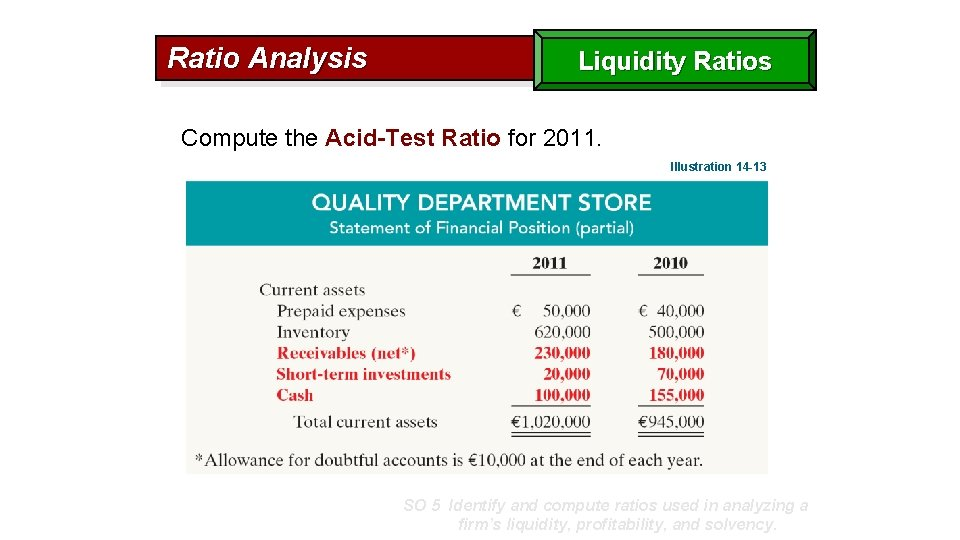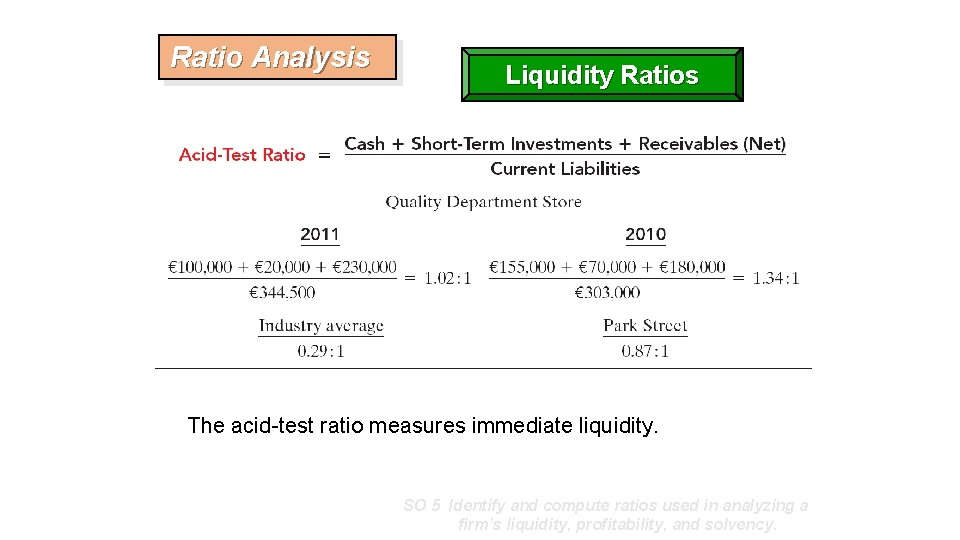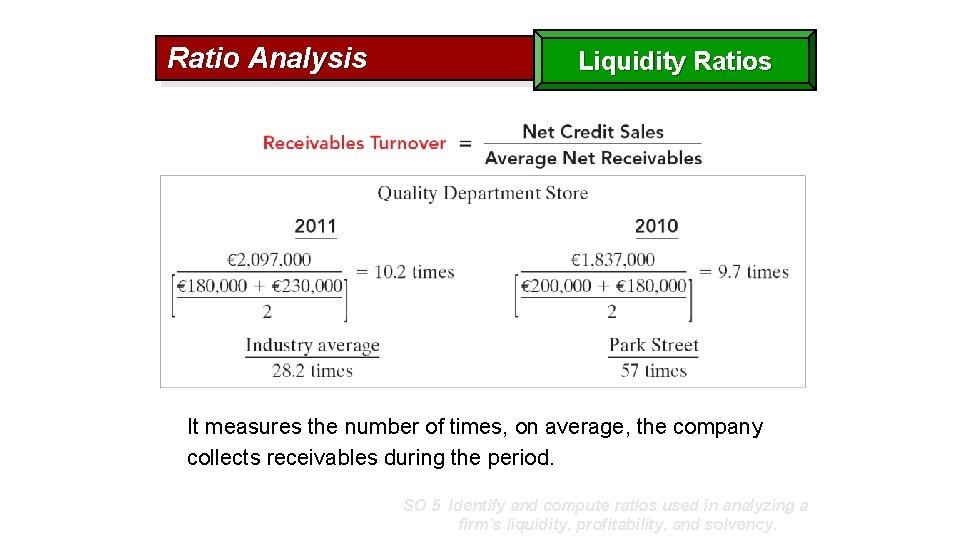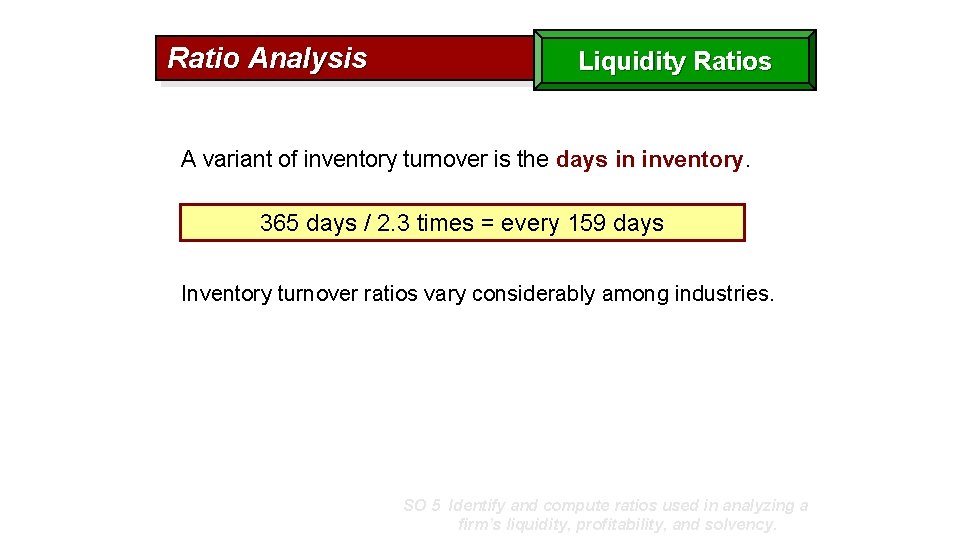# Liquidity Ratios Ratio Analysis Liquidity Ratios Compute the

• Slides: 8Liquidity RatiosRatio Analysis Liquidity Ratios Compute the Acid-Test Ratio for 2011. Illustration 14 -13 SO 5 Identify and compute ratios used in analyzing a firm’s liquidity, profitability, and solvency.Ratio Analysis Liquidity Ratios The acid-test ratio measures immediate liquidity. SO 5 Identify and compute ratios used in analyzing a firm’s liquidity, profitability, and solvency.Ratio Analysis Liquidity Ratios It measures the number of times, on average, the company collects receivables during the period. SO 5 Identify and compute ratios used in analyzing a firm’s liquidity, profitability, and solvency.Ratio Analysis Liquidity Ratios A variant of the receivables turnover ratio is to convert it to an average collection period in terms of days. 365 days / 10. 2 times = every 35. 78 days This means that receivables are collected on average every 36 days. SO 5 Identify and compute ratios used in analyzing a firm’s liquidity, profitability, and solvency.Ratio Analysis Liquidity Ratios Inventory turnover measures the number of times, on average, the inventory is sold during the period. SO 5 Identify and compute ratios used in analyzing a firm’s liquidity, profitability, and solvency.Ratio Analysis Liquidity Ratios A variant of inventory turnover is the days in inventory. 365 days / 2. 3 times = every 159 days Inventory turnover ratios vary considerably among industries. SO 5 Identify and compute ratios used in analyzing a firm’s liquidity, profitability, and solvency.Ratio Analysis Liquidity Ratios Illustration 14 -27 Summary of liquidity ratios SO 5 Identify and compute ratios used in analyzing a firm’s liquidity, profitability, and solvency.